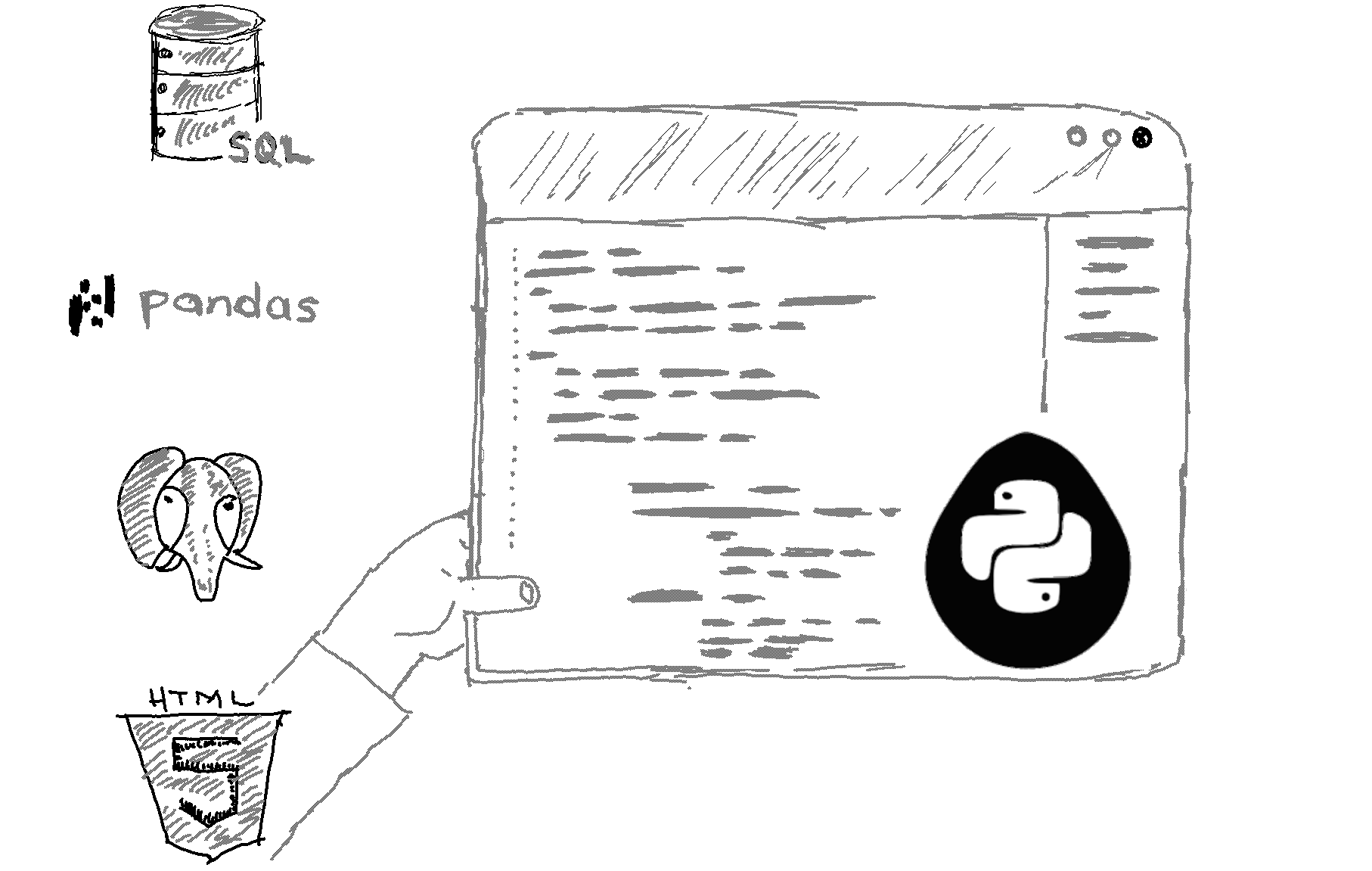# Here is how to get the current time in Python.

```import datetime

# Get the current time
now = datetime.datetime.now()

# Print the current time
print(now) ```

Output
This will print something like '2022-12-14 18:32:15.372267', which is the current date and time.
Explanation

To get the current time in Python, you can use the datetime module's datetime.now() method. This method returns a datetime object that represents the current date and time.

If you only want to get the current time, without the date, you can use the time() method instead of the now() method. This method returns a time object that represents the current time, without the date. Here's an example:

```import datetime

# Get the current time
now = datetime.time.time()

# Print the current time
print(now)```
This will print something like '18:32:15.372267', which is the current time without the date.

Note that the time is returned in the local time zone, unless you specify a different time zone using the tzinfo argument of the time() method. For example, to get the current time in the UTC time zone, you can use the following code:

```import datetime

# Get the current time in the UTC time zone
now = datetime.time.time(tzinfo=datetime.timezone.utc)

# Print the current time
print(now)```
This will print the current time in the UTC time zone.

Related HowTos
##### Recommended Course

Learn Flask development and learn to build cool apps with our premium Python course on Udemy.